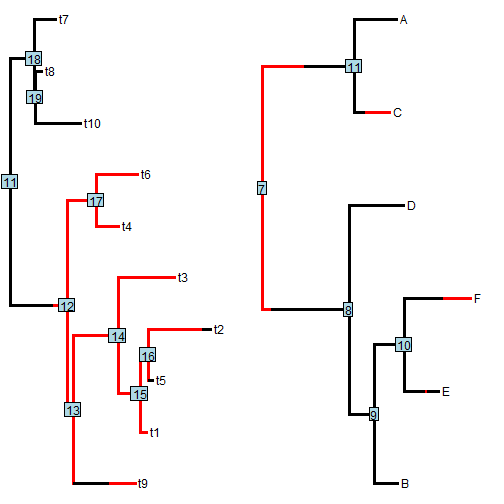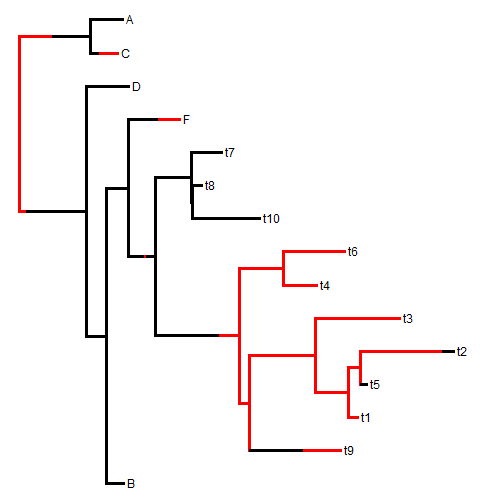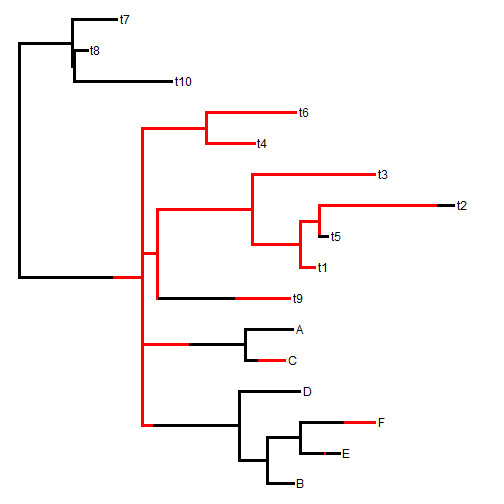## Friday, May 20, 2016

### Basic `bind.tree.simmap` in phytools

Contrary to prior statements, I have now pushed `bind.tree.simmap` (from yesterday's blog post) into phytools on GitHub (1, 2).

So, for instance, given two trees with mapped discrete characters of class `"simmap"`:

``````library(phytools)
t1
``````
``````##
## Phylogenetic tree with 10 tips and 9 internal nodes.
##
## Tip labels:
##  t9, t1, t5, t2, t3, t4, ...
##
## The tree includes a mapped, 2-state discrete character with states:
##  a, b
##
## Rooted; includes branch lengths.
``````
``````t2
``````
``````##
## Phylogenetic tree with 6 tips and 5 internal nodes.
##
## Tip labels:
##  "B" "E" "F" "D" "C" "A"
##
## The tree includes a mapped, 2-state discrete character with states:
##  a, b
##
## Rooted; includes branch lengths.
``````
``````par(mfcol=c(1,2))
plot(t1,lwd=3)
``````
``````## no colors provided. using the following legend:
##       a       b
## "black"   "red"
``````
``````nodelabels()
plot(t2,lwd=3)
``````
``````## no colors provided. using the following legend:
##       a       b
## "black"   "red"
``````
``````nodelabels()
``````we can bind `t1` onto `t2` as follows:

``````t3<-bind.tree.simmap(t2,t1,where=which(t2\$tip.label=="E"))
plot(t3,lwd=3)
``````
``````## no colors provided. using the following legend:
##       a       b
## "black"   "red"
``````or, of course, we can bind `t2` to `t1`, for instance:

``````t4<-bind.tree.simmap(t1,t2,where=12)
plot(t4,lwd=3)
``````
``````## no colors provided. using the following legend:
##       a       b
## "black"   "red"
``````As of yet there are no features to add along an edge or with a root edge, but at some point I'll figure that out too.

This version of phytools can be installed from GitHub as follows:

``````library(devtools)
install_github("liamrevell/phytools")
``````